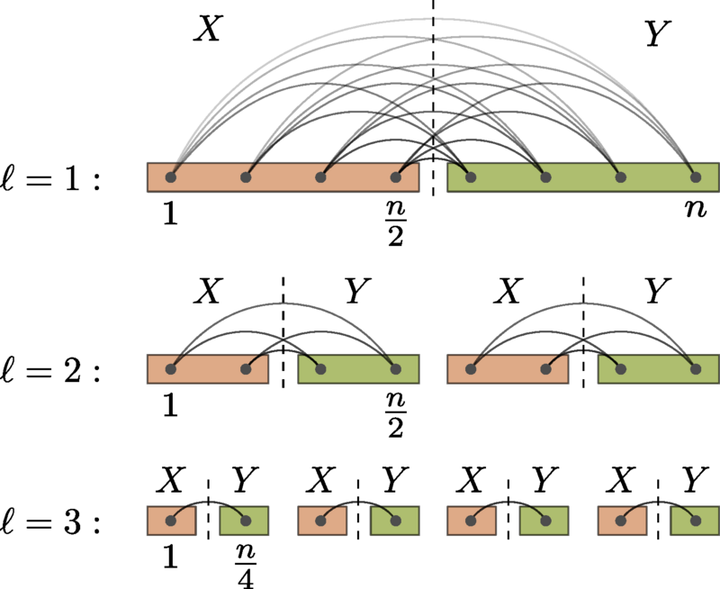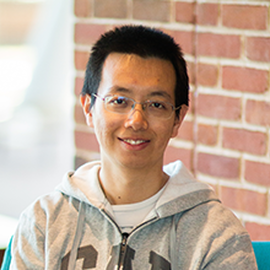# On the complexity of implementing Trotter stepsImage credit: Minh C. Tran

### Abstract

Quantum dynamics can be simulated on a quantum computer by exponentiating elementary terms from the Hamiltonian in a sequential manner. However, such an implementation of Trotter steps has gate complexity depending on the total Hamiltonian term number, comparing unfavorably to algorithms using more advanced techniques.

We develop methods to perform faster Trotter steps with gate complexity sublinear in the number of terms. We focus on a class of $2$-local Hamiltonians in one spatial dimension whose interaction strength decays with distance $x$ according to power law $1/x^\alpha$ ($\alpha>0$), although we discuss extensions to higher spatial dimensions as well. Naively, Trotter steps for $n$-qubit power-law interactions require $\Omega(n^2)$ gates to implement.

Our first method is based on block encodings of power-law systems with efficiently computable coefficients. While block encodings typically slow down quantum simulation by a factor proportional to the $1$-norm of Hamiltonian coefficients, we overcome this barrier using a recursive Trotter decomposition to effectively reduce the norm. We obtain further improvements by simulating commuting Hamiltonian terms with an average combination cost. The resulting complexity is almost linear in the spacetime volume for $\alpha\geq 2$ and improves the state of the art for $\alpha<2$.

We also show that Trotter steps can be implemented more efficiently when certain blocks of Hamiltonian coefficients exhibit low-rank properties. In particular, using a recursive low-rank decomposition, we show that power-law Hamiltonians can be simulated with gate complexity nearly linear in the spacetime volume for all $\alpha\geq1$.

We apply our methods to simulate electronic structure Hamiltonians in second quantization in real space. Combining with a tighter error analysis, we show that it suffices to use $\left(\eta^{1/3}n^{1/3}+\frac{n^{2/3}}{\eta^{2/3}}\right)n^{1+o(1)}$ gates and $\mathcal{O}\left(\log(n)\right)$ ancilla qubits to simulate uniform electron gas in real space with $n$ spin orbitals and $\eta$ electrons, asymptotically improving the best results from previous work. We obtain an analogous result when the external potential of nuclei is introduced under the Born-Oppenheimer approximation.

We prove a circuit lower bound when the Hamiltonian coefficients take a continuum range of values. Specifically, we construct a class of $2$-local Hamiltonians with commuting terms that requires at least $\Omega(n^2)$ gates to evolve with accuracy $\epsilon=\Omega(1/\mathrm{poly}(n))$ for time $t=\Omega(\epsilon)$. Our proof is based on a gate-efficient reduction from the approximate synthesis of diagonal unitaries within the Hamming weight-$2$ subspace, which may be of independent interest.

Our result thus suggests the use of Hamiltonian structural properties as both necessary and sufficient to implement Trotter steps with lower gate complexity.

Type
Publication
PRX Quantum 4, 020323##### Yuan Su
###### Senior Researcher

I work on quantum algorithms for simulating Hamiltonian dynamics. I am particularly interested in the design, analysis, implementation, and application of quantum simulation.